## Filters

Sort by :
Clear All
Q
Engineering
1262 Views   |

Two straight long conductors AOB and COD are perpendicular to each other and carry currents i1 and i2. The magnitude of the magnetic induction at a point P at a distance a from the point O in a direction perpendicular to the plane ACBD is

• Option 1)

• Option 2)

• Option 3)

• Option 4)

As we learnt in  For Infinite Length - - wherein   Magnetic field due to both AOB and COD are respectively :  and  are perpendicular to each other. Option 1) This option is incorrect. Option 2) This option is incorrect. Option 3) This option is correct. Option 4) This option is incorrect.
Engineering
232 Views   |

Two long wires are hanging freely. They are joined first in parallel and then in series and then are connected with a battery. In both cases, which type of force acts between the two wires

• Option 1)

Attraction force when in parallel and repulsion force when in series

• Option 2)

Repulsion force when in parallel and attraction force when in series

• Option 3)

Repulsion force in both cases

• Option 4)

Attraction force in both cases

As we learnt in  Force between two parallel current carrying conductors - - wherein I1 and I2 current carrying two parallel wires  a-seperation between two wires    When they are connected in parallel the current flowing through both are in same direction hence they attract each other. when they are in parallel they will tend to repel each other Option 1)  Attraction force when in parallel...
Engineering
469 Views   |

Two coaxial solenoids 1 and 2 of the same length are set so that one is inside the other. The number of turns per unit length are n1 and  n2. The currents  i1 and  i2 are flowing in opposite directions. The magnetic field inside the inner coil is zero. This is possible when

• Option 1)

i1≠i2    and    n1=n2

• Option 2)

i1=i2    and     n1≠n2

• Option 3)

i1=i2    and     n1=n2

• Option 4)

i1n1=i2n2

As we learnt in  Magnetic field due to Infinite length solenoid -  Bin= - wherein Point is well inside the solenoid    For          Option 1)  i1≠i2    and    n1=n2   incorrect Option 2) i1=i2    and     n1≠n2   incorrect Option 3)  i1=i2    and     n1=n2   correct Option 4)  i1n1=i2n2   incorrect
Engineering
616 Views   |

H+, He+ and O++ ions having same kinetic energy pass through a region of space filled with uniform magnetic field B directed perpendicular to the velocity of ions. The masses of the ions H+, He+ and O++ are respectively in the ratio 1:4:16. As a result

• Option 1)

H+ ions will be deflected most

• Option 2)

O++ ions will be deflected least

• Option 3)

He+ and O++ ions will suffer same deflection

• Option 4)

All ions will suffer the same deflection

As we learnt in  Radius of charged particle - -   Deflection  Ratio of deflection:   Therefore deflection is same for He+ and O++ . Option 1) H+ ions will be deflected most   incorrect Option 2) O++ ions will be deflected least   incorrect Option 3) He+ and O++ ions will suffer same deflection   correct Option 4) All ions will suffer the same deflection incorrect
Engineering
258 Views   |

current carrying loop is placed in a uniform magnetic field in four different orientations, I,II, III & IV arrange them in the decreasing order of potential Energy

I.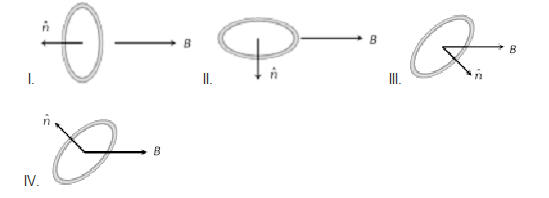• Option 1)

I > III > II > IV

• Option 2)

I > II >III > IV

• Option 3)

I > IV > II > III

• Option 4)

III > IV > I > II

As we learnt in  Potential energy of current carrying coil - -    Potential  energy  (I) angle between  &  is 180; U = MB (II)angle between  &  is 90;U = 0 (III) angle between M & B is  ( < 90o), U = -MB cos  (IV) angle between M & B is (>90o), U = + MB cos       I > IV > II > III Option 1)  I > III > II > IV     incorrect Option 2)  I > II >III > IV   incorrect Option 3)  I > IV > II >...
Engineering
452 Views   |

An ionized gas contains both positive and negative ions. If it is subjected simultaneously to an electric field along the +x direction and a magnetic field along the +z direction, then

• Option 1)

Positive ions deflect towards +y direction and negative ions towards -y direction

• Option 2)

All ions deflect towards +y direction

• Option 3)

All ions deflect towards -y direction

• Option 4)

Positive ions deflect towards -y direction and negative ions towards +y direction

As we learnt in  Force on a current carrying conductor - - wherein idl=current element    Due to electric field positive ion will move along +x direction and -ve ion along -x direction. Since magnetic field is along +z direction, it will apply force in -y direction for both positive and negative ion. Option 1) Positive ions deflect towards +y direction and negative ions towards -y...
Engineering
516 Views   |

A thin circular wire carrying a current I has a magnetic moment M. The shape of the wire is changed to a square and it carries the same current. It will have a magnetic moment

• Option 1)

M

• Option 2)

• Option 3)

• Option 4)

Magnetic moment (M) - M=NiA - wherein N-number of turns in the coil  i-current throughout the coil  A-area of the coil    Initial Final                              Option 1) M   incorrect Option 2)   incorrect Option 3)   incorrect Option 4) correct
Engineering
136 Views   |

A particle of mass m and charge q moves with a constant velocity v along the positive x direction. It enters a region containing a uniform magnetic field B directed along the negative z direction, extending from x = a to x = b. The minimum value of v required so that the particle can just enter the region  x>b  is

• Option 1)

• Option 2)

• Option 3)

• Option 4)

As we learnt in  Radius of charged particle - - For particle to enter x > b Radius  (b - a)    or        Option 1) incorrect Option 2) correct Option 3) incorrect Option 4) incorrect
Engineering
395 Views   |

A particle of charge q and mass m moves in a circular orbit of radius r with angular speed ω. The ratio of the magnitude of its magnetic moment to that of its angular momentum depends on

• Option 1)

ω and q

• Option 2)

ω, q and m

• Option 3)

q and m

• Option 4)

ω and m

As we learnt in  Magnetic moment (M) - M=NiA - wherein N-number of turns in the coil  i-current throughout the coil  A-area of the coil    Magnetic moment   angular momentum Option 1) ω and q   incorrect Option 2) ω, q and m   incorrect Option 3) q and m   correct Option 4) ω and m   incorrect
Engineering
90 Views   |

A long straight wire along the z-axis carries a current I in the negative z direction. The magnetic vector field   at a point having coordinates (x, y) in the z = 0 plane is

• Option 1)

• Option 2)

• Option 3)

• Option 4)

As we learnt in  For Infinite Length - - wherein   Magnitude of magnetic field Direction of field along tangential direction Option 1)   correct Option 2) incorrect Option 3) incorrect Option 4)   incorrect
Engineering
169 Views   |

A horizontal rod of mass 10 gm and length 10 cm is placed on a smooth plane inclined at an angle of 60 degree with the horizontal, with the length of the rod parallel to the edge of the inclined plane. A uniform magnetic field of induction B is applied vertically downwards. If the current through the rod is 1.73 ampere, then the value of B for which the rod remains stationary on the inclined plane is

• Option 1)

1.73 Tesla

• Option 2)

1 / 1.73Tesla

• Option 3)

1 Tesla

• Option 4)

None of the above

As we learnt in Total magnetic force - - wherein       For equilibrium mg sin  = i l B cos        B = 1 T Option 1) 1.73 Tesla   incorrect  Option 2) 1 / 1.73Tesla incorrect  Option 3) 1 Tesla   correct  Option 4) None of the above incorrect
Engineering
693 Views   |

A conducting loop carrying a current I is placed in a uniform magnetic field pointing into the plane of the paper as shown. The loop will have a tendency to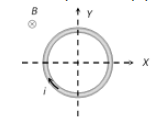• Option 1)

Contract

• Option 2)

Expand

• Option 3)

Move towards +ve x -axis

• Option 4)

Move towards ?ve x-axis

As we learnt in  Force on a current carrying conductor - - wherein idl=current element   Consider small portion of coil. Force acting on this portion is radially outward. Hence the tendency of coil is to expand. Option 1) Contract   incorrect Option 2) Expand   correct Option 3) Move towards +ve x -axis   incorrect Option 4) Move towards ?ve x-axis incorrect
Engineering
328 Views   |

A coil having N turns is wound tightly in the form of a spiral with inner and outer radii a and b respectively. When a current I passes through the coil, the magnetic field at the centre is

• Option 1)

• Option 2)

• Option 3)

• Option 4)

As we learnt in  Magnetic Field due to Circular Current at the centre - If a coil of radius r is carrying current i then magnetic feild at a distnace X from its centre is: - wherein    Consider a small thickness dx at distance x from centre. Number of turn in   Option 1)       incorrect Option 2)   incorrect Option 3)   correct Option 4) incorrect
Engineering
119 Views   |

A circular current–carrying coil has a radius R. The distance from the centre of the coil, on the axis, where B will be 1/8 of its value at the centre of the coil is

• Option 1)

• Option 2)

• Option 3)

• Option 4)

As we learnt in  Magnetic Field at the axis due to circular current carrying wire - - wherein N is numbers of turn in coil          8R3 = (R2 + x2)3/2  4R2 = R2 + x2  x =  R Option 1) incorrect Option 2) correct Option 3) incorrect Option 4) incorrect
Engineering
826 Views   |

A cell is connected between the points A and C of a circular conductor ABCD of centre O with angle AOC=60o If B1 and B2 are the magnitudes of the magnetic fields at O due to the currents in ABC and ADC respectively, the ratio B1/B2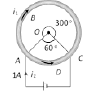• Option 1)

0.2

• Option 2)

6

• Option 3)

1

• Option 4)

5

As we learnt in  Arc subtends angle theta at the centre - - wherein           Option 1) 0.2 incorrect Option 2) 6 incorrect Option 3) 1 correct Option 4) 5 incorrect
Engineering
281 Views   |

A and B are two conductors carrying a current i in the same direction. x and y are two electron beams moving in the same direction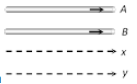• Option 1)

There will be repulsion between A and B attraction between x and y

• Option 2)

There will be attraction between A and B, repulsion between x and y

• Option 3)

There will be repulsion between A and B and also x and y

• Option 4)

There will be attraction between A and B and also x and y

As we learnt in  force between two parallel current carrying conductors - - wherein I1 and I2 current carrying two parallel wires  a-seperation between two wires    Two parallel current attract each other and hence A and B will attract each other. e- & e- will repel each other as these two are similar charges. Option 1) There will be repulsion between A and B attraction between x and...
Engineering
1083 Views   |

A wire of length L metre carrying a current of I ampere is bent in the form of a circle. Its magnitude of magnetic moment will be

• Option 1)

• Option 2)

• Option 3)

• Option 4)

As we learnt in  Magnetic moment (M) - M=NiA - wherein N-number of turns in the coil  i-current throughout the coil  A-area of the coil     Magnetic moment  Option 1)   incorrect  Option 2) correct  Option 3) incorrect  Option 4) incorrect
Engineering
570 Views   |

A non-planar loop of conducting wire carrying a current I is placed as shown in the figure. Each of the straight sections of the loop is of length 2a. The magnetic field due to this loop at the point P (a,0,a) points in direction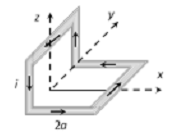• Option 1)

1/√2(−j^+k^)

• Option 2)

1/√3(−j^+k^+i^)

• Option 3)

1/√3(i^+j^+k^)

• Option 4)

1/√2(i^+k^)

As we learnt in Magnetic Field due to Circular Current at the centre - If a coil of radius r is carrying current i then magnetic feild at a distnace X from its centre is: - wherein Magnetic field due to horizontal loop is in  and magnetic field due to vertical loop is along  . So the resultant field is  Resultant Direction is   Option 1)  1/√2(−j^+k^)   incorrect Option...
Exams
Articles
Questions